« Exercise 4.9.6 Seismic behaviour » Exercise 4.9.7 Given : Thickness of slabs s1, s2, s3, s4, h=160 mm, s6: h=210 mm and covering load gεπ=2.0 kN/m2. Question : Perform static analysis to determine the distribution of slabs loads transferred onto beams.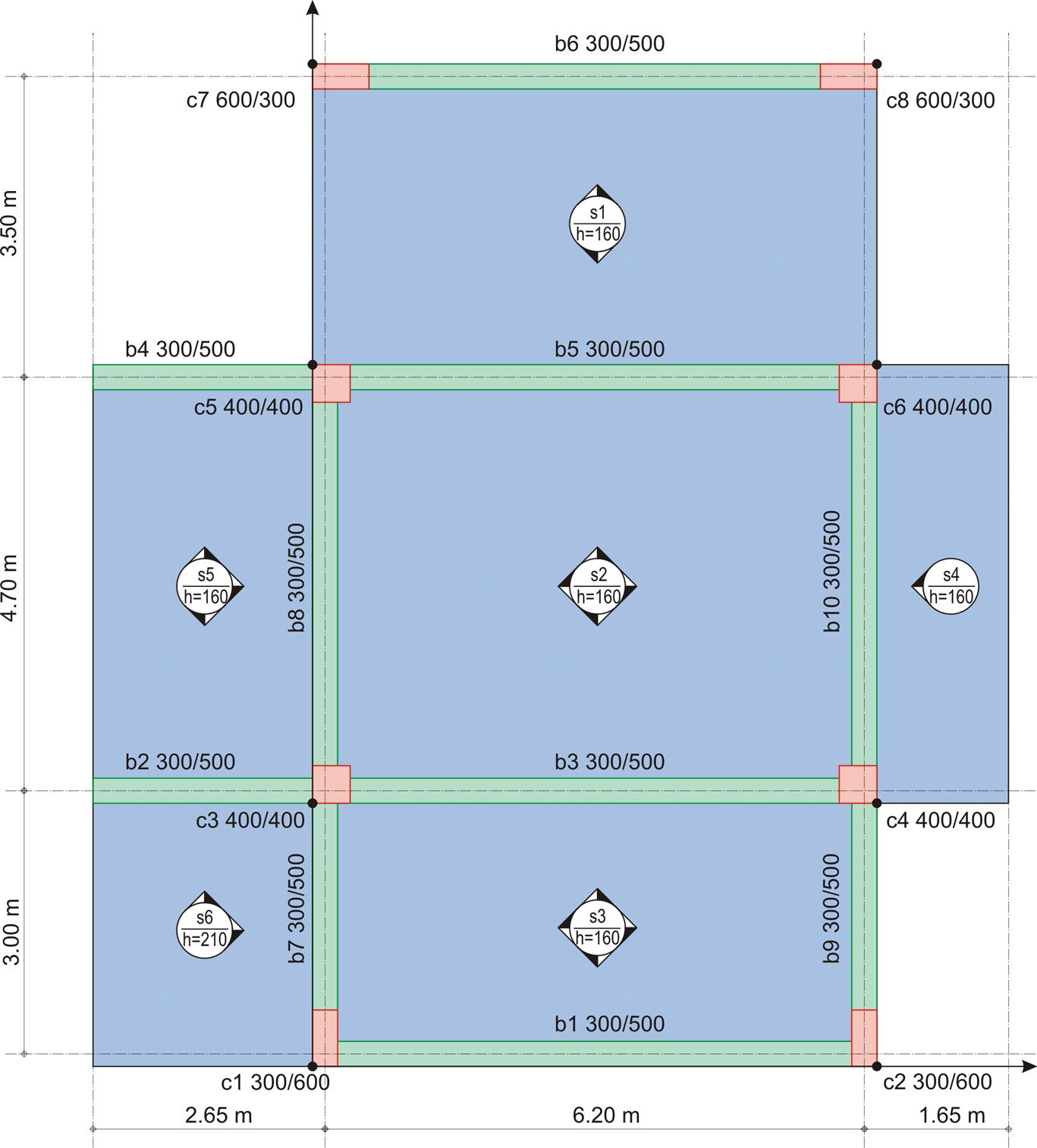Figure 4.9.7-1 Figure 4.9.7-1 Static analysis g1,2,3,4,5=0.16x1.0x25.0=4.0 kN/m2, g6=0.21x1.0x25.0=5.25 kN/m2, gεπ=2.0 kN/m2 Analysis will be performed using the global load (due to the small value of the live load): p1,2,3,4,5=6.0x1.35+2.0x1.5=11.1 kN/m2, p6=7.25x1.35+2.0x1.5=12.80 kN/m2 Thus, on 1.0 m wide strips, the respective loads are p1, 2,3,4,5=11.1 kN/m and p6=12.80 kN/m. Slab s1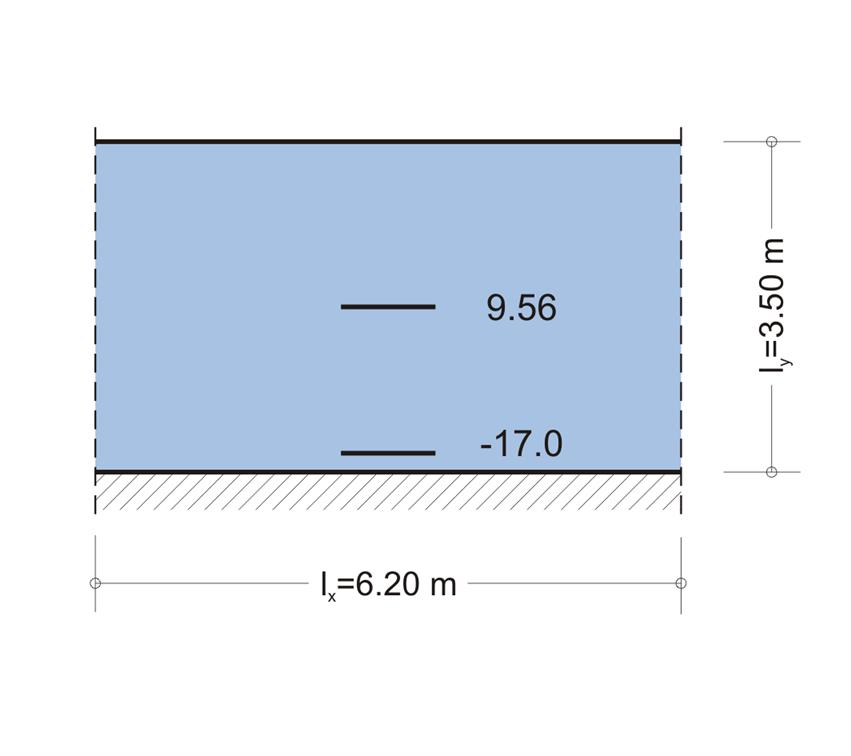Figure 4.9.7-2 Figure 4.9.7-2 The slab is fixed at one edge: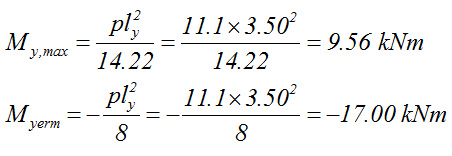Slab s2 From table 50-2, by switching indices x, y, we get: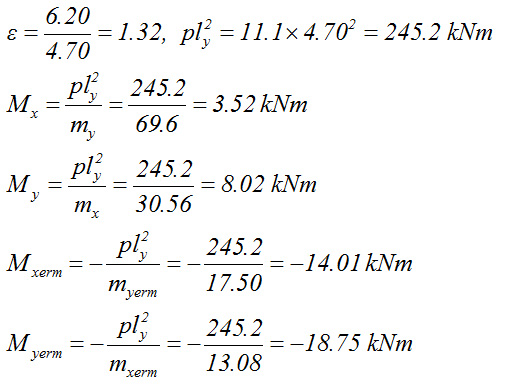Slab s3 From table 49, by switching indices x, y, we get: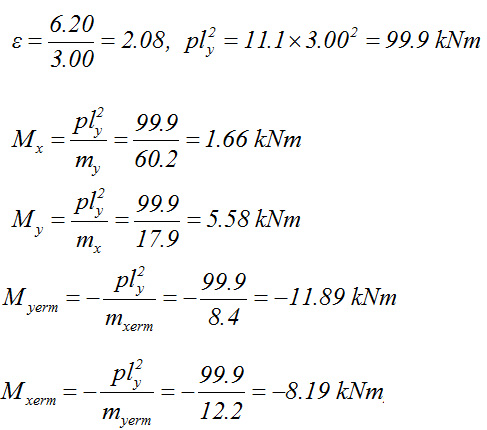Slab s4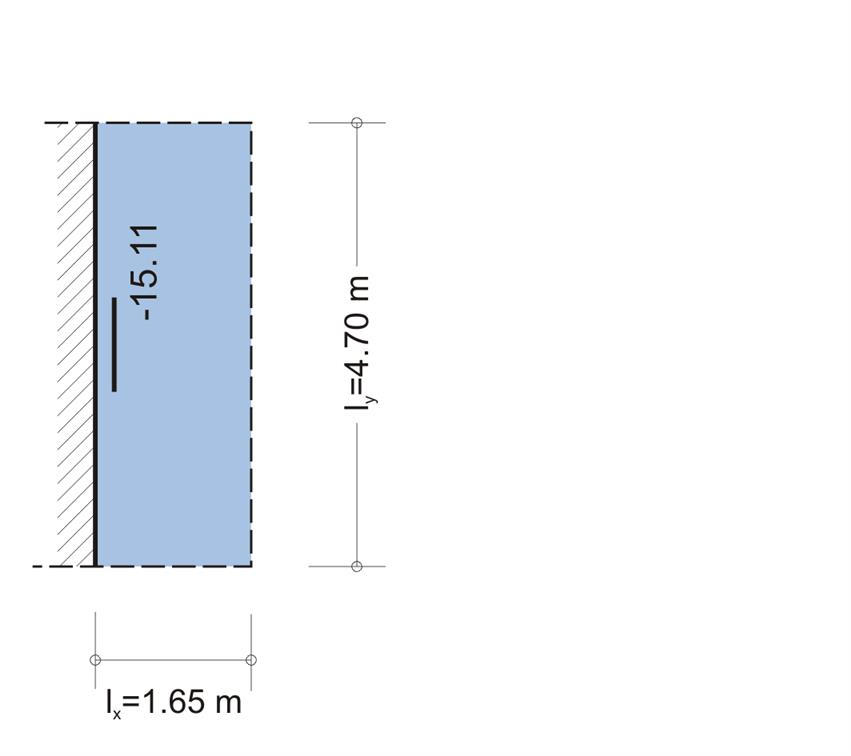Figure 4.9.7-5 Figure 4.9.7-5 s4 is a cantilever slab: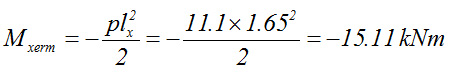Slab s5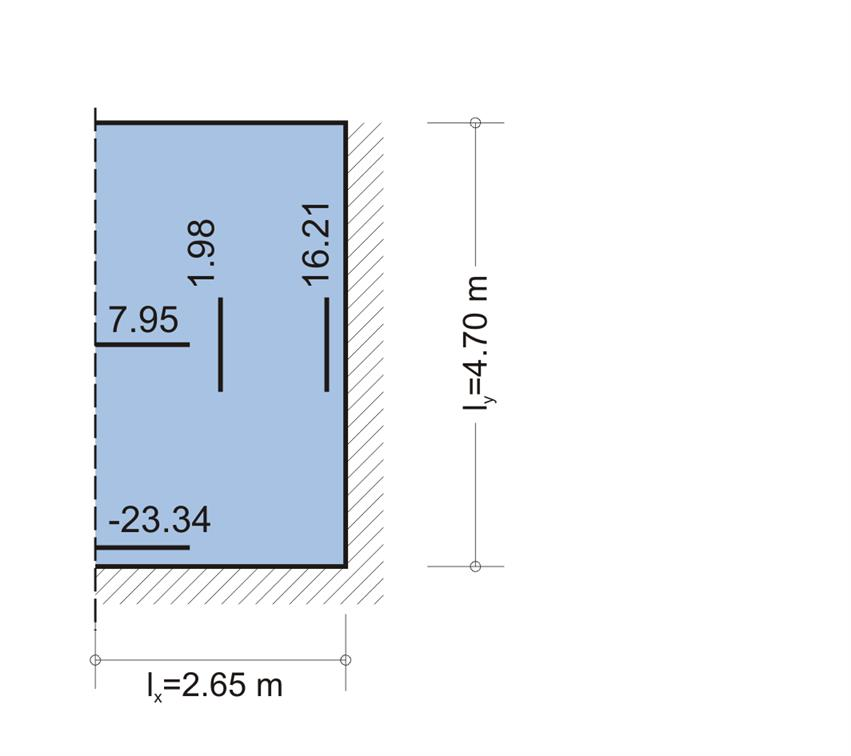Figure 4.9.7-6 Figure 4.9.7-6 The slab is supported on three edges. From table 55, by switching indices x, y, we get: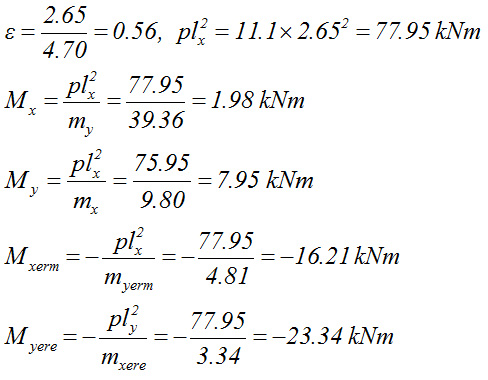Slab s6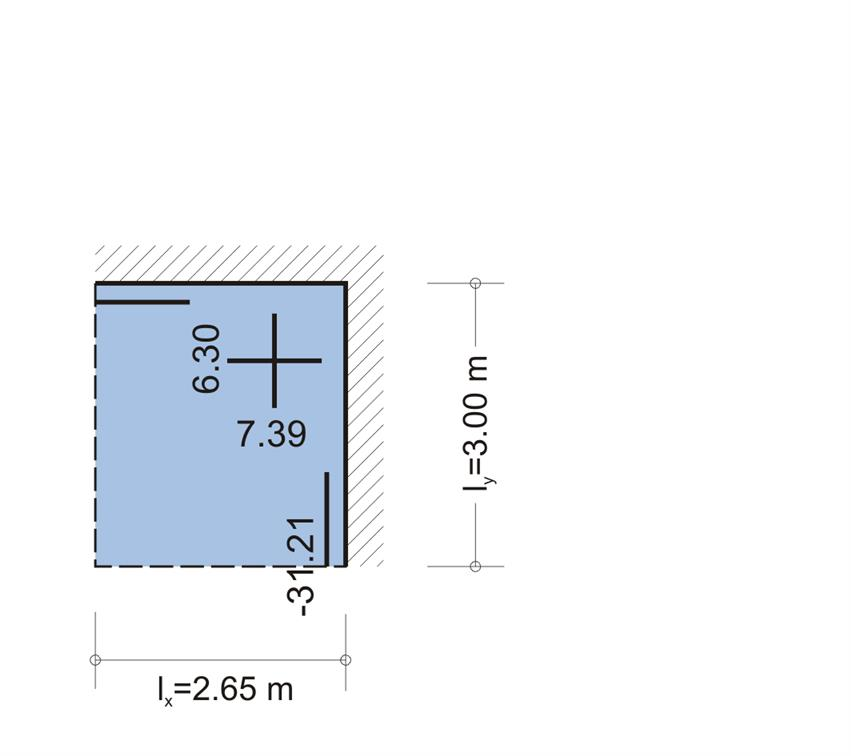Figure 4.9.7-7 Figure 4.9.7-7 The slab is supported on two adjacent edges. From table 59 we get: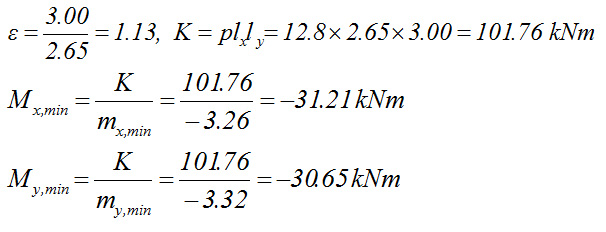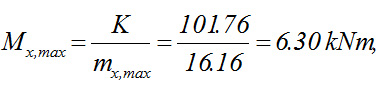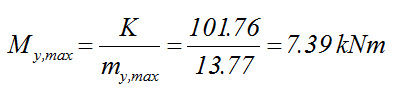Supports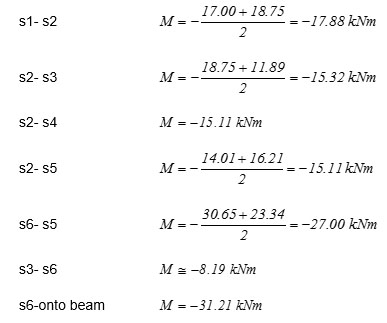Final stress resultants Notes: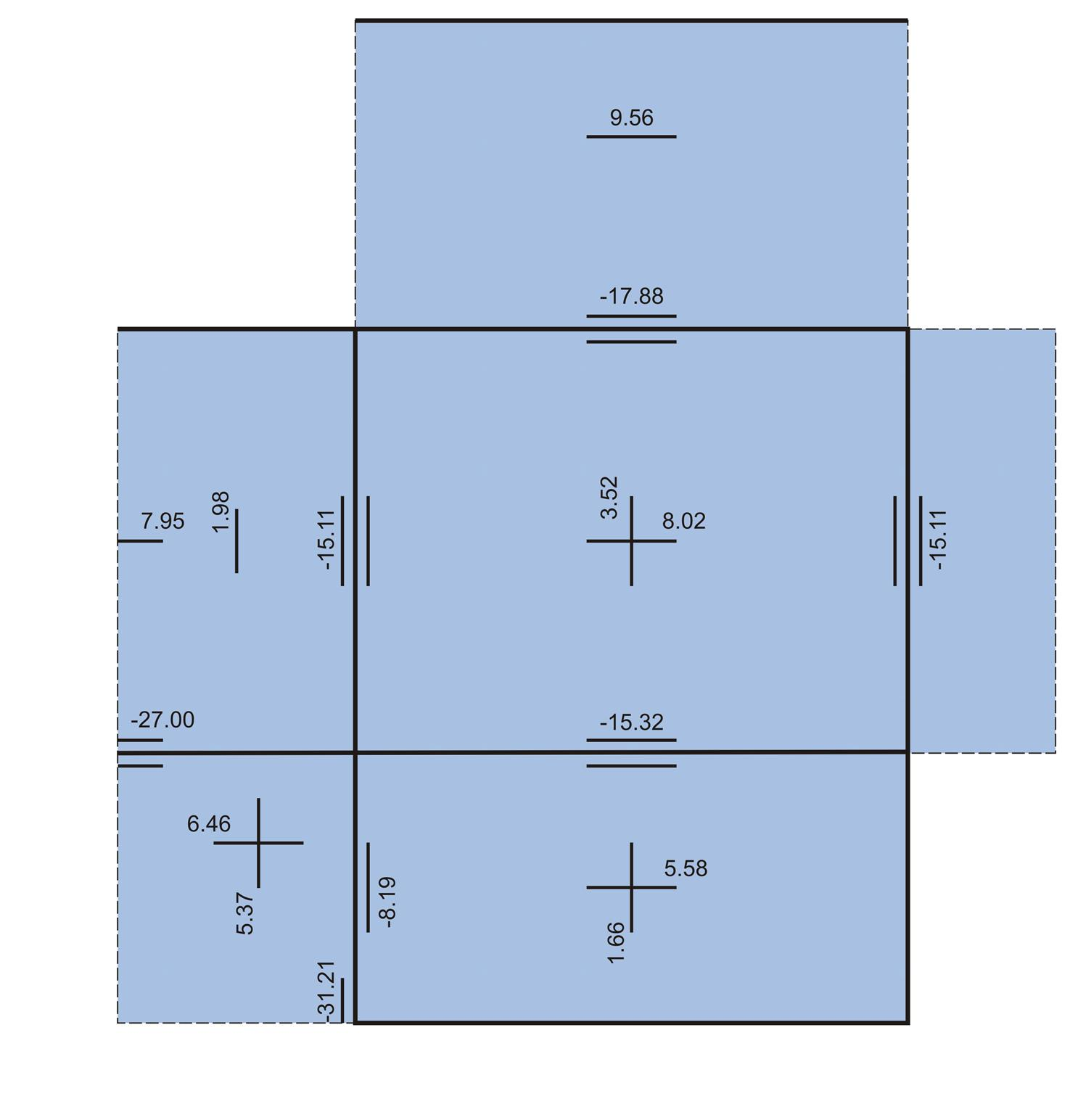Figure 4.9.7-11 Figure 4.9.7-11 · In general, the most unfavourable span moments, arise in one-way slabs (s1) or cantilever slabs (s4) at one point in the first direction and at one line in the other. In two-way slabs moments are developed at the same point in both directions. · The moment peak values decrease rapidly with the distance from the peak, while at the fixed supports of slabs supported on three or two adjacent edges the decrease is quite rapid. · The moment at the support s5-s6 or at the beam support of s6, in the middle of its 1.00 m wide strip, where the design calculations will be performed, may be much lesser. To this end, the reinforcement calculated in these supports will decrease significantly with the distance from supports. Slabs load distribution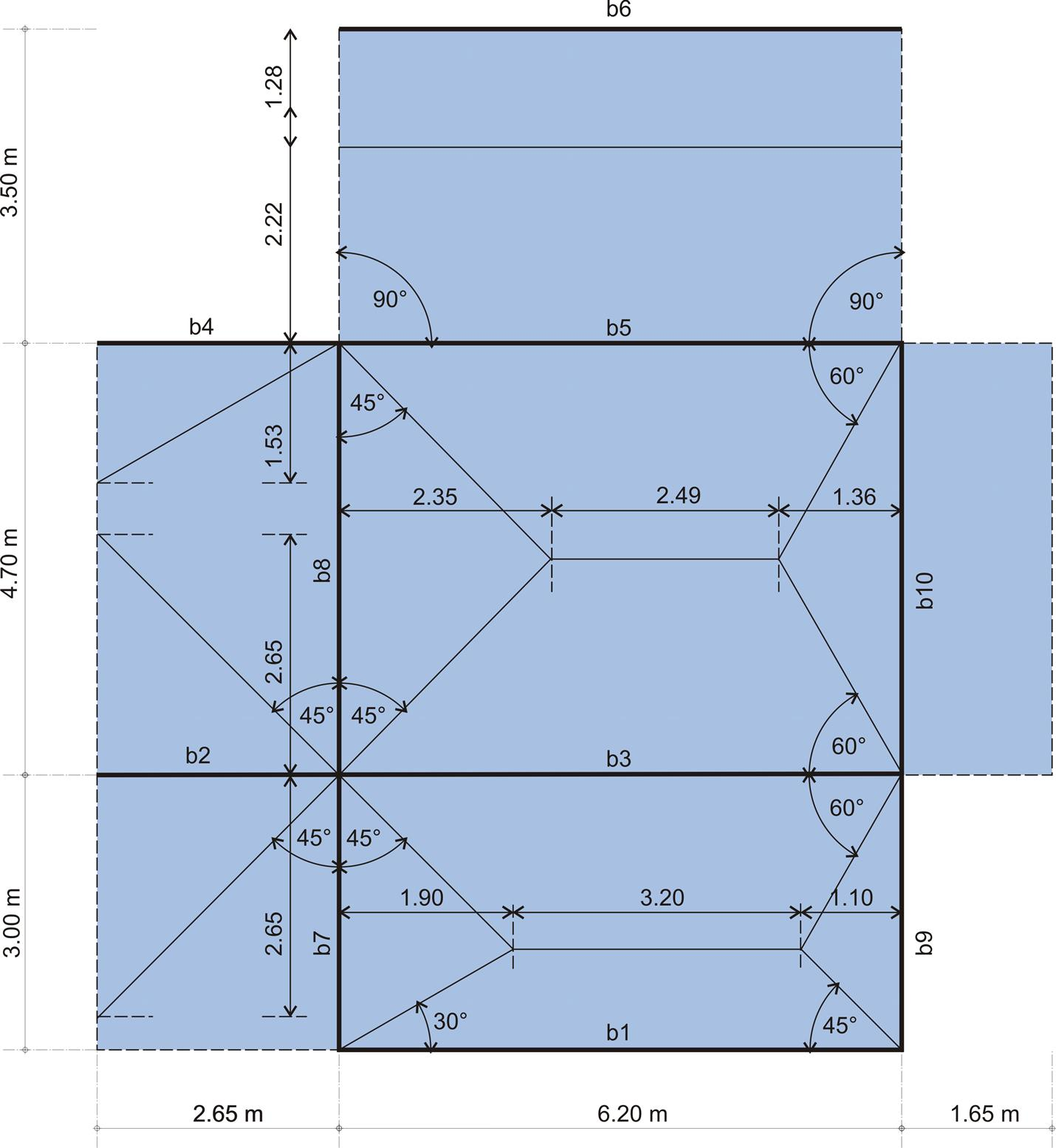Figure 4.9.7-9 Figure 4.9.7-9 Note: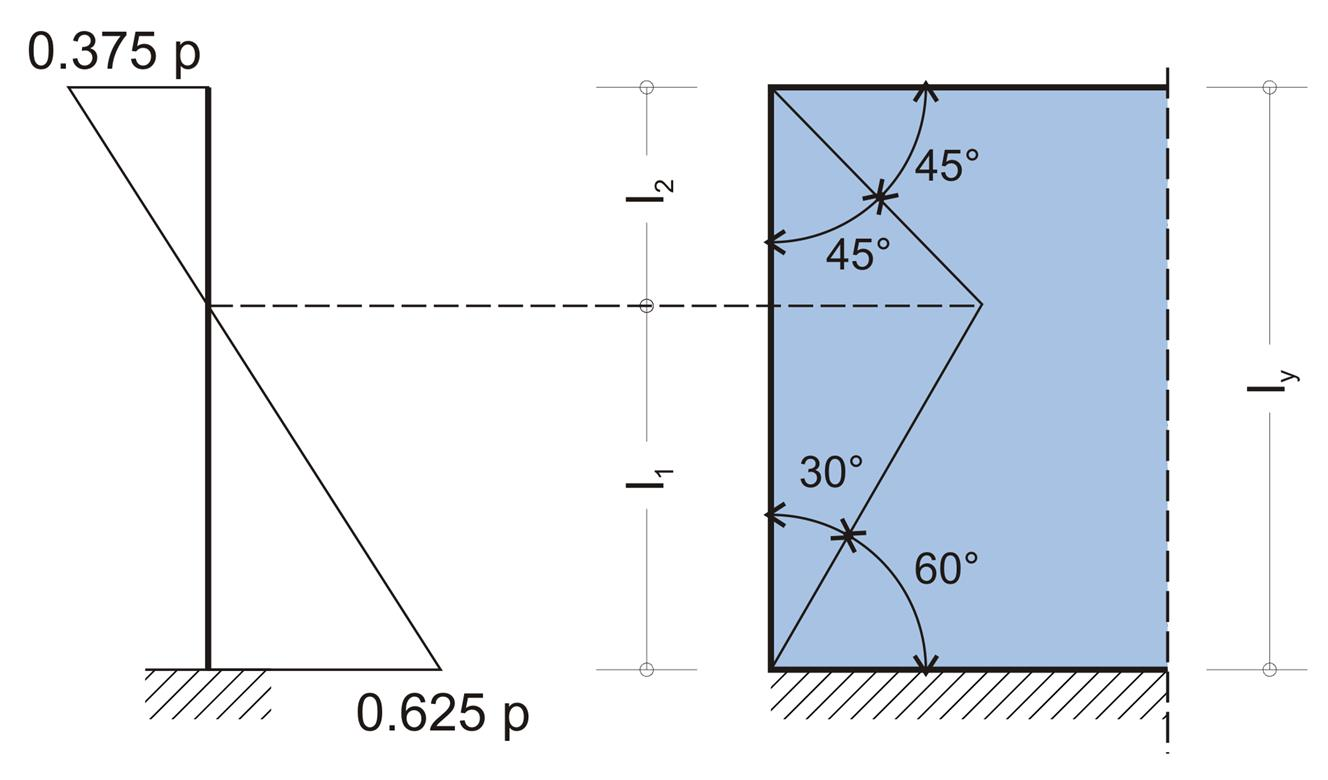Figure 4.9.7-10 Figure 4.9.7-10 Regarding slab s1 and assuming load distribution at an angle of 45ο or 60ο we have: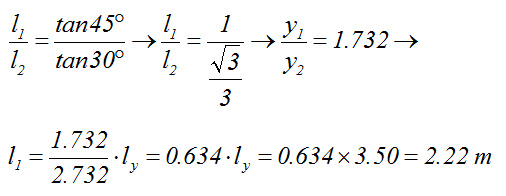For a bar fixed at one end the resulted quotient is: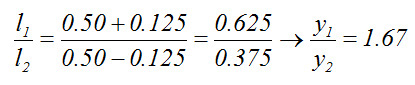which is almost the same with the quotient resulted from the assumption of angles. Slab loads are distributed onto beams according to their influence region. The loading (kN/m) at each point of the beam is equal to the load ( kN/m2) multiplied by the respective abscissa (m). Example : Loads on beams b4, b5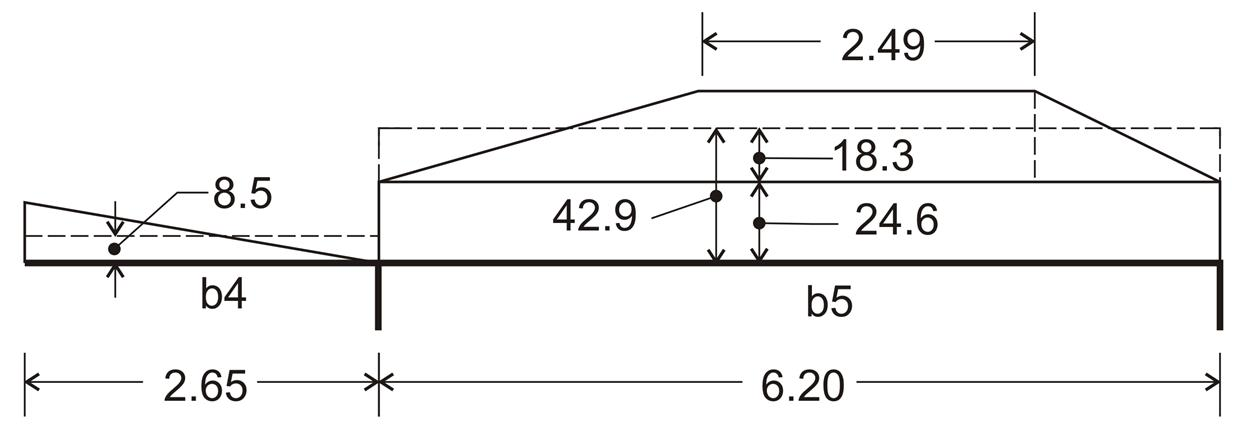Figure 4.9.7-11 Figure 4.9.7-11 Load on beam b4: The triangular influence region of slab s5 load has an area of 1.53x2.65/2=2.03 m 2 resulting to load of 2.03m 2 x11.1kN/m 2 =22.53 kN and eventually to equivalent uniform load of 22.53kN/2.65m= 8.5 kN/m . Load on beam b5: From slab s1: due to the rectangular influence region, equivalent uniform load directly results to 2.22mx11.1kN/m2=24.6 kN/m. From slab s2: trapezoidal influence region has an area of (6.20+2.49)x2.35/2=10.21 m2 which gives load of 10.21m2x11.1kN/m 2=113.33kN and finally equivalent uniform load of 113.33kN/6.20m=18.3 kN/m. Overall: equivalent uniform load of beam b5 = 24.6 + 18.3=42.9 kN/m Equivalent uniform loadings are obtained directly by tables. For example, from table 50-2 for the distribution of load transferred from slab s2 onto b5, it yields: pyerm=υxerm · p · ly =0.35x11.1x4.70=18.3 kΝ/m, while from table 55 for the distribution of load transferred from slab s5 onto b4, it yields: pyr=υxr · p x l· =0.29x11.1x2.65=8.5 kΝ/m.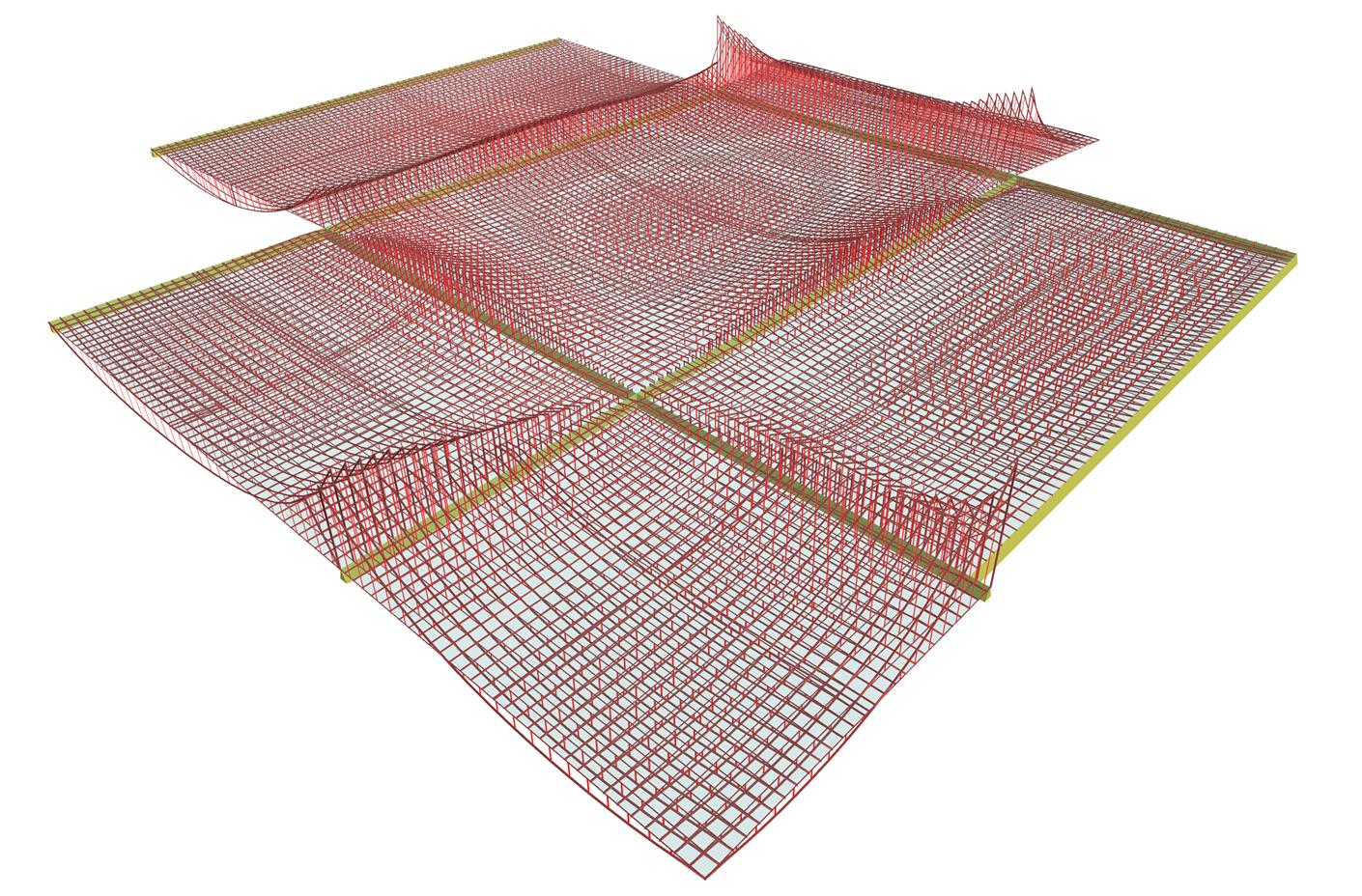Figure 4.9.7-12: Bending moment distribution, produced by the related software Figure 4.9.7-12: Bending moment distribution, produced by the related software « Exercise 4.9.6 Seismic behaviour »